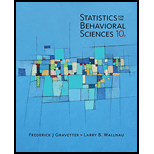Chapter 3, Problem 7P### Statistics for The Behavioral Scie...

10th Edition
Frederick J Gravetter + 1 other
ISBN: 9781305504912

#### Solutions

Chapter
Section### Statistics for The Behavioral Scie...

10th Edition
Frederick J Gravetter + 1 other
ISBN: 9781305504912
Textbook Problem

# A sample of n = 8 scores has a mean of M = 12. What is the value of EX for this sample?

To determine

Find X for the given sample.

Explanation

Given:

A sample of n=8 scores have a mean of M=12 .

Formula used:

X =M×n

Calculations:

The mean is the average of scores. To find mean, add all scores and divide the sum by number of scores in the sample. Therefore:

M= X n

### Still sussing out bartleby?

Check out a sample textbook solution.

See a sample solution

#### The Solution to Your Study Problems

Bartleby provides explanations to thousands of textbook problems written by our experts, many with advanced degrees!

Get Started

## Additional Math Solutions

#### Evaluate the integral, if it exists. 11sinx1+x2dx

Single Variable Calculus: Early Transcendentals, Volume I

#### Change 20 qt to gallons.

Elementary Technical Mathematics

#### For y = (x3 + 1)2, y = _____. a) 2x(x3 + 1) b) 6x(x3 + 1) c) 6x2(x3 + 1) d) 3x2(x3 + 1)

Study Guide for Stewart's Single Variable Calculus: Early Transcendentals, 8th

#### The graph of is: a) b) c) d)

Study Guide for Stewart's Multivariable Calculus, 8th